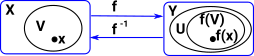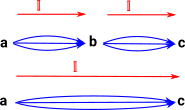# Maths - Continuity

#### Definition 1

Here is one definition of continuity, based on open sets:

 Let X and Y be topological spaces. A function f : X->Y is continuous if f-1(V) is open for every open set V in Y.#### Definition 2

Another definition of continuity, based on neighbourhoods, is equivilant to the above definition.

 Let X and Y be topological spaces. A function f : X->Y is continuous if for every xX and every open set U containing f(x), there exists a neighbourhood V of x such that f(V)U.## Fibrations

### Homotopy Lifting Property

https://en.wikipedia.org/wiki/Homotopy_lifting_property

## Transport

 from here `transport : Path U A B -> A -> B`

That is, if we have a path from A to B and A, then B.

## Composition of Paths

 We want to compose these two paths: p : Path A a b q : Path A b cfrom here```compPath (A : U) (a b c : A) (p : Path A a b) (q : Path A b c) : Path A a c = comp (<_> A) (p @ i) [ (i = 0) -> a , (i = 1) -> q ]``` Where 'comp' is a keyword with the following parameters: a path in the universe. the bottom of the cube we are computing. a list of the sides of the cube

 metadata block see also: Michael Robinson - Youtube from two-day short course on Applied Sheaf Theory: Correspondence about this page Book Shop - Further reading . Where I can, I have put links to Amazon for books that are relevant to the subject, click on the appropriate country flag to get more details of the book or to buy it from them.Introduction to Topological Manifolds (Graduate Texts in Mathematics S.) Other Books about Curves and Surfaces

This site may have errors. Don't use for critical systems.